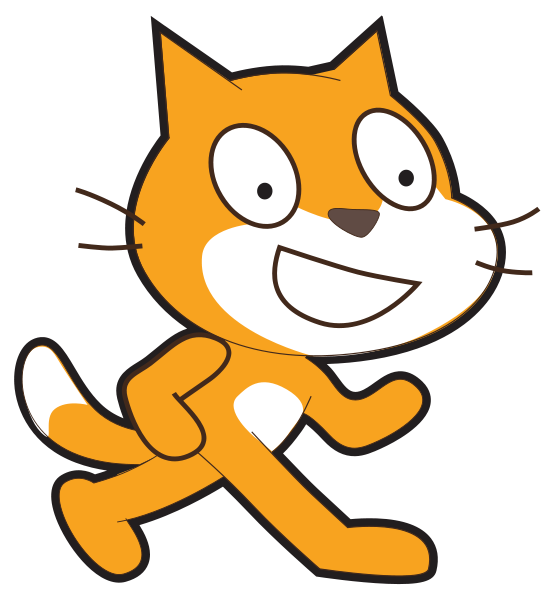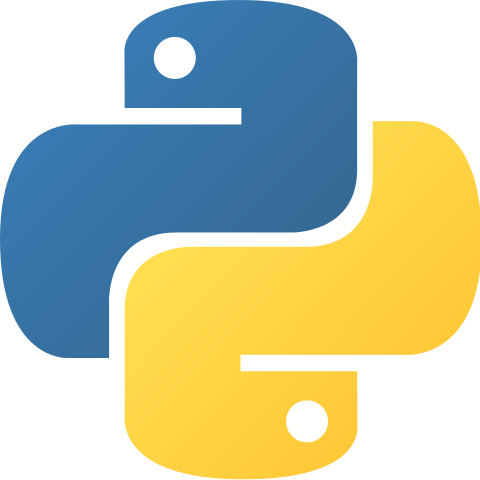# Display Binary Numbers (using variables as an operator)

Challenge Level: Beginner

Learning outcomesStudents will be able to:

## Requirement:

Write a program to display numbers 1, 2, 4, 8 and 16 on the screen one at a time. This is similar to the previous challenge, but instead of storing a number directly into the variable, you should double the value in the variable each time. You need to use an operator (for multiplying 2 numbers) for this challenge.

## Testing examples:

The output will always show the numbers 1, 2, 4, 8 and 16 (there is no input).

## Languages

Scratch#### What it should look like

Click on the green flag to see the expected output of your program.

Recommended blocks
when flag clicked
set [number of dots v] to 

set [number of dots v] to ((number of dots) * (2))

set [number of dots v] to ((number of dots) * (2))

set [number of dots v] to ((number of dots) * (2))

set [number of dots v] to ((number of dots) * (2))
say (number of dots) for (1) secs

say (number of dots) for (1) secs

say (number of dots) for (1) secs

say (number of dots) for (1) secs

say (number of dots) for (1) secs
Hints
• Make a variable called “number of dots” and change its value by using an operator (multiplying it by 2 before displaying it on the screen each time). You can find “make a new variable” under “data” script to create a new variable. Make sure your variable name is as descriptive as possible.
• Use the set [number of dots v] to  block to set the value of your new variable. Use the () * () operation under “Operators” to double the value of your variable.
• Display the value of your variable on the screen by replacing “Hello” in your say [Hello] for (2) secs block with your new variable.
PythonBlock-based## Extra Challenge

Display the numbers from largest to smallest (display the numbers 16, 8, 4, 2 and 1).